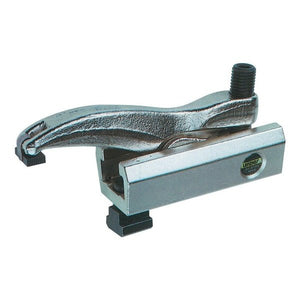## Categories# Lenzke Clamp MQ100 (to 60kN)

‚Ä¢ Patented Stepless Adjustment
‚Ä¢ Strong Clamping pressure for small clamping torque
‚Ä¢ Flexible and adjustable at every angle to the T slot
‚Ä¢ Reduction of set-up time through reliable, fast and safe clamping
‚Ä¢ Vertically highly adjustable through triple clamping shoe
‚Ä¢ Widely adjustable clamping heights through stackable clamping shoe

Thread Slot a H Comments Part no Price £ Quantity
M12 14 0-48mm V=82, L=95, L1=12, L2=20, B=52, b=24, h=37, kN=32 100-048-1214 999.00 - +
M12 14 5-68mm V=112, L=135, L1=12, L2=28, B=52, b=24, h=37, kN=30 100-067-1214 999.00 - +
M16 18 0-55mm V=95, L=110, L1=15, L2=25, B=60, b=28, h=42, kN=40 100-055-1618 999.00 - +
M16 18 6-80mm V=135, L=155, L1=15, L2=32, B=60, b=28, h=42, kN=43 100-078-1618 260.72 - +
M20 22 0-62mm V=110, L=125, L1=19, L2=32, B=75, b=34, h=48, kN=45 100-062-2022 999.00 - +
M20 22 7-90mm V=159, L=175, L1=19, L2=38, B=75, b=34, h=48, kN=49 100-088-2022 999.00 - +
M24 28 8-80mm V=125, L=150, L1=22, L2=38, B=88, b=42, h=57, kN=55 100-077-2428 425.22 - +
M24 28 8-105mm V=182, L=205, L1=22, L2=45, B=88, b=42, h=57, kN=60 100-100-2428 460.35 - +
M30 36 8-80mm V=128, L=150, L1=25, L2=-, B=88, b=42, h=57, kN=55 100-077-3036 350.40 - +
M30 36 8-105mm V=185, L=205, L1=25, L2=45, B=88, b=42, h=57, kN=60 100-100-3036 468.00 - +